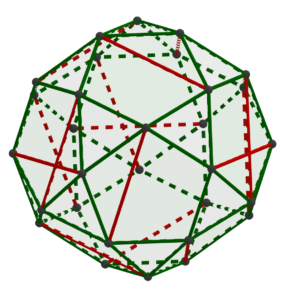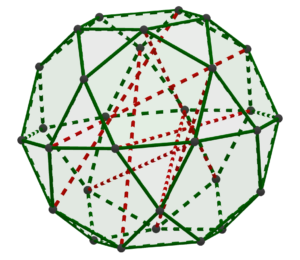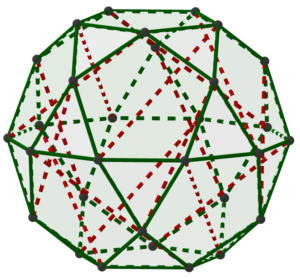07 Nov

When I set the goal of creating a new tensegrity structure for the Storm King Art Center workshop, I decided that I wanted to create a highly regular, symmetric structure, to contrast with the more free-form, organic style of Snelson’s Free Ride Home at the Center. (Snelson also created many very regular tensegrity sculptures himself.)

As is often the case, the creative process began with an existing structure that shared many of the characteristics I wanted, namely the classic six-strut tensegrity structure. In a previous article, we saw how that structure is based on a regular icosahedron; in fact, the compression members are just six diagonals of an icosahedron that are equivalent under the symmetries of the icosahedron. Moreover, the end points of the diagonals exhaust the twelve vertices of the icosahedron.

Thus, it seemed that I might obtain a different interesting structure if I started with a different polyhedron that shared some key properties with the icosahedron, and then looked for an appropriate set of diagonals. So the first question was, what are those key properties of the icosahedron? Well, we want all of the edges of the shape to be the “same” in some sense so that when we fabricate them from tension members with the same tension, they will balance each other uniformly. And what does “same” mean in this context? Well, a natural answer is that there should be some symmetry of the shape — in other words, some shape-preserving transformation, which in this case will be a rotation — which will match any edge up with any other edge. When the symmetries of an object completely scramble some component of that object, mathematicians call the object “transitive” for that component. So I could find a list of candidate shapes by an internet search for “edge-transitive polyhedron.” That search turned up a fancy synonym for edge-transitive, namely “isotoxal,” and it also turned up this Wikipedia page, of which the first table is of particular interest.

The rhombic triacontahedron immediately caught my eye. However, it includes two different categories of vertices, the ones where five edges meet and the ones where three edges meet; and what’s worse, there are twelve of the former but twenty of the latter. That means there will be no way to make sixteen equivalent diagonals, since some of them will not terminate at a five-edge vertex at either end, while others will.

So instead, I set my sights on it’s left-hand neighbor in that table, the icosidodecahedron. It has 30 identical vertices (consistent with our theme, the symmetries of the icosidodecahedron act transitively on the vertices). So then I searched for a set of fifteen diagonals of the icosidodecahedron, all the same length, to be the struts of the new tensegrity.

I began with what seemed like the simplest sort of diagonal, namely a diagonal between two vertices that are only “two edges apart,” i.e., both adjacent to the same vertex. I was able to draw a set of diagonals that exhausted the vertices, like this:However, there’s a problem: you can see on the right-hand side of the image that two of the diagonals selected intersect. While that’s OK mathematically, there’s no way to direct struts to pass through each other. And try as I might, I couldn’t avoid two of these diagonals intersecting. From a math point of view, the natural response was to try to prove that any such set of diagonals contains (at least) two that intersect each other. That turns out to be the case, and here’s how the proof goes: Any diagonal that connects two vertices that are adjacent to the same vertex is actually a diagonal of one of the pentagonal faces of the icosidodecahedron. There are only twelve such faces, but we need 15 diagonals. Hence, at least one of the faces will end up with two diagonals. And (as you can demonstrate for yourself by drawing some diagrams) any two diagonals of a regular pentagon intersect.

So these “simplest” diagonals were out. And it also occurred to me that for building a tensegrity structure, we likely want long diagonals that go through the body of the polyhedron, to make it easier for the tension of the rubber bands along the edges of the polyhedron to pull against them. The very longest diagonals of the icosidodecahedron all intersect each other at the center of the polyhedron, so they’re out. Therefore, my next attempt was to use the second-longest diagonals, like this partial effort:You can see that this selection of diagonals already has intersecting pairs. In fact, I was not able to find any selection of diagonals that fit the requirements above, leading to this Math StackExchange question. So what did I do? I settled for the “near miss” below. The ten diagonals that connect a “polar” vertex to an “equatorial” vertex are transitive, but there’s no way to transform them to one of the other five diagonals (that connect two “mid-latitude” vertices, one above the equator and one below.) In fact, the two types of diagonals are different lengths, with the first type about 20% longer than the second. So I couldn’t expect the resulting tensegrity structure to come out regular, using struts the same length for diagonals that should theoretically be the same length. But I hoped it would at least be close.So this image became the template for building the new tensegrity structure, which you can read about in the next MakeStream post.